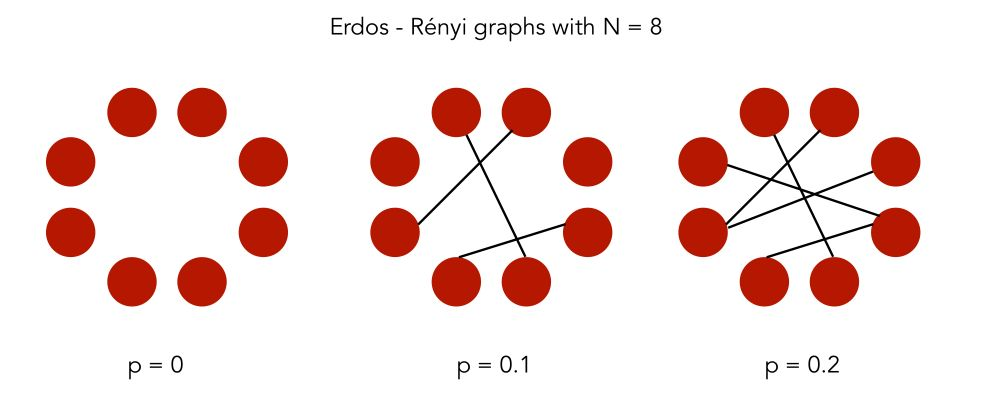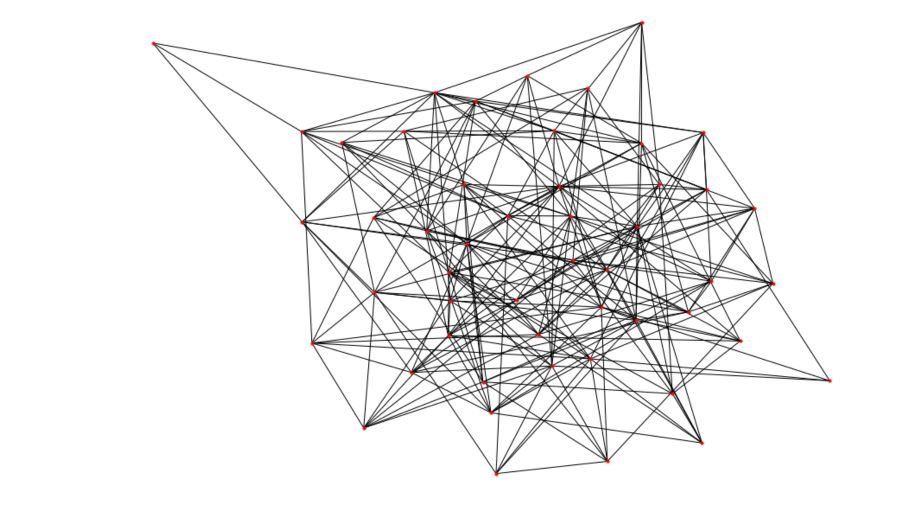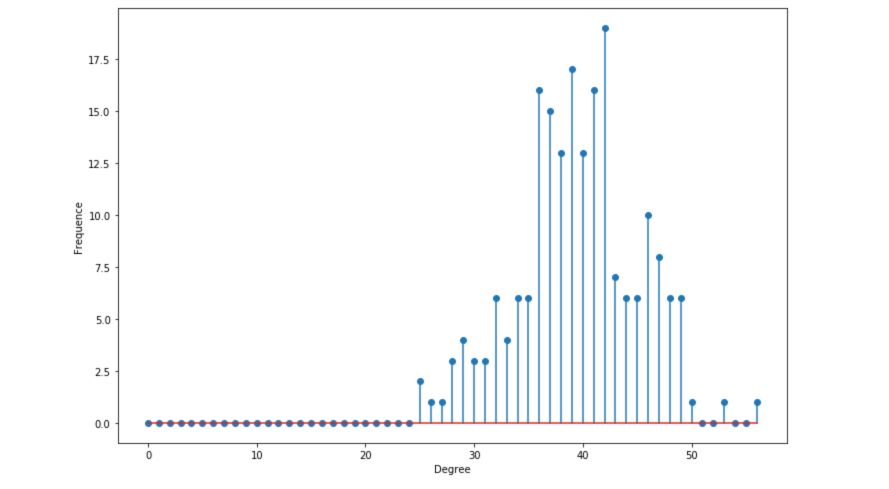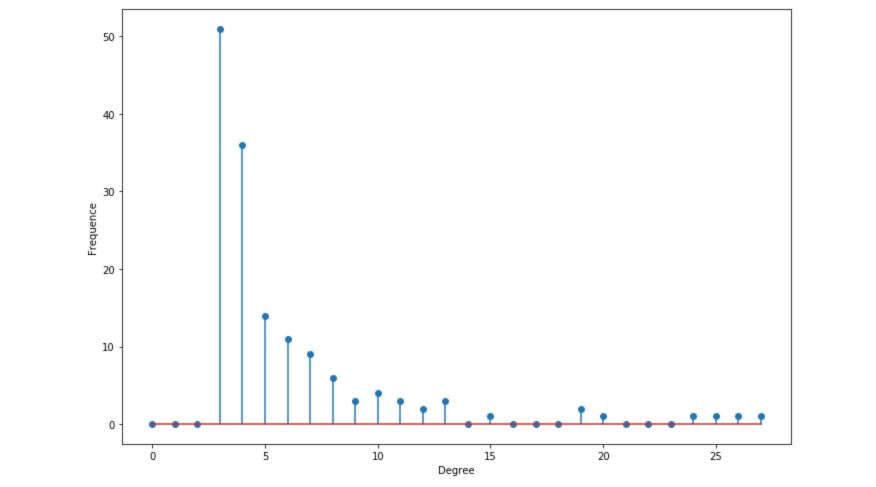Let’s now dig deeper into graph analysis! What are the main kinds of graphs? What are their properties?

## Introduction

For what comes next, open a Jupyter Notebook and import the following packages :

import numpy as np
import random
import networkx as nx
from IPython.display import Image
import matplotlib.pyplot as plt


If you have not already installed the networkx package, simply run :

pip install networkx


The following articles will be using the latest version 2.x of networkx. NetworkX is a Python package for the creation, manipulation, and study of the structure, dynamics, and functions of complex networks.

We can analyze a graph at different scales :

• using the global properties of the network
• using communities and clusters
• or by looking at individual nodes

The main descriptive measures we’ll explore will be :

• the degree distribution
• the clustering coefficient
• the “small world” phenomena
• the centrality of a node
• the diameter of the graph …

We’ll now cover the most common types of graph models :

## Erdos-Rényi model

### Definition

In an Erdos-Rényi model, we build a random graph model with $$n$$ nodes. The graph is generated by drawing an edge between a pair of nodes $$(i,j)$$ independently with probability $$p$$. We, therefore, have 2 parameters: $$n$$ the number of nodes and $$p$$In Python, the networkx package has a built-in function to generate Erdos-Rényi graphs.

# Generate the graph
n = 50
p = 0.2
G_erdos = nx.erdos_renyi_graph(n,p, seed =100)

# Plot the graph
plt.figure(figsize=(12,8))
nx.draw(G_erdos, node_size=10)


You’ll get a result pretty similar to this one :### Degree distribution

Let $$p_k$$ the probability that a randomly selected node has a degree $$k$$. Due to the random way the graphs are built, the distribution of the degrees of the graph is binomial :

$p_k = {n-1 \choose k} p^k (1-p)^{n-1-k}$

The distribution of the number of degrees per node should be close to the mean. The probability of high nodes decreases exponentially.

degree_freq = np.array(nx.degree_histogram(G_erdos)).astype('float')

plt.figure(figsize=(12, 8))
plt.stem(degree_freq)
plt.ylabel("Frequence")
plt.xlabel("Degree")
plt.show()


To visualize the distribution, I have increased $$n$$ to 200.### Descriptive statistics

• The average degree is given by $$n \times p$$. With $$p = 0.2$$ and $$n = 200$$, we are centered around 40.
• The degree expectation is given by $$(n-1) \times p$$
• The maximum degree is concentrated around the average
# Get the list of the degrees
degree_sequence_erdos = list(G_erdos.degree())

nb_nodes = n
nb_arr = len(G_erdos.edges())

avg_degree = np.mean(np.array(degree_sequence_erdos)[:,1])
med_degree = np.median(np.array(degree_sequence_erdos)[:,1])

max_degree = max(np.array(degree_sequence_erdos)[:,1])
min_degree = np.min(np.array(degree_sequence_erdos)[:,1])

esp_degree = (n-1)*p

print("Number of nodes : " + str(nb_nodes))
print("Number of edges : " + str(nb_arr))

print("Maximum degree : " + str(max_degree))
print("Minimum degree : " + str(min_degree))

print("Average degree : " + str(avg_degree))
print("Expected degree : " + str(esp_degree))
print("Median degree : " + str(med_degree))


This should give you something similar to :

Number of nodes: 200
Number of edges: 3949
Maximum degree: 56
Minimum degree: 25
Average degree: 39.49
Expected degree: 39.800000000000004
Median degree: 39.5


The average and the expected degrees are close since there is only a small factor between the two.

## Barabasi-Albert model

### Definition

In a Barabasi-Albert model, we build a random graph model with $$n$$ nodes. The graph is generated by the following algorithm :

• Step 1: With a probability $$p$$, move to the second step. Else, move to the third step.
• Step 2: Connect a new node to existing nodes chosen uniformly at random
• Step 3: Connect the new node to $$n$$ existing nodes with a probability proportional to their degree

Such a graph aims to model preferential attachment, which is often observed in real networks.

In Python, the networkx package has also a built-in function to generate Barabasi-Albert graphs.

# Generate the graph
n = 150
m = 3
G_barabasi = nx.barabasi_albert_graph(n,m)

# Plot the graph
plt.figure(figsize=(12,8))
nx.draw(G_barabasi, node_size=10)


You’ll get a result pretty similar to this one :You can easily notice how some nodes appear to have a much larger degree than others now!

### Degree distribution

Let $$p_k$$ the probability that a randomly selected node has a degree $$k$$. The degree distribution follows a power law :

$p_k \propto k^{-\alpha}$

The distribution is now heavy-tailed. There is a large number of nodes that have a small degree, but a significant number of nodes have a high degree.

degree_freq = np.array(nx.degree_histogram(G_barabasi)).astype('float')

plt.figure(figsize=(12, 8))
plt.stem(degree_freq)
plt.ylabel("Frequence")
plt.xlabel("Degree")
plt.show()The distribution is said to be scale-free, in the sense that the average degree is not informative.

### Descriptive statistics

• The average degree is constant if $$\alpha ≤ 2$$, else, it diverges
• The maximum degree is $$O(n^{ \frac {1} { \alpha -1}})$$
# Get the list of the degrees
degree_sequence_erdos = list(G_erdos.degree())

nb_nodes = n
nb_arr = len(G_erdos.edges())

avg_degree = np.mean(np.array(degree_sequence_erdos)[:,1])
med_degree = np.median(np.array(degree_sequence_erdos)[:,1])

max_degree = max(np.array(degree_sequence_erdos)[:,1])
min_degree = np.min(np.array(degree_sequence_erdos)[:,1])

esp_degree = (n-1)*p

print("Number of nodes : " + str(nb_nodes))
print("Number of edges : " + str(nb_arr))

print("Maximum degree : " + str(max_degree))
print("Minimum degree : " + str(min_degree))

print("Average degree : " + str(avg_degree))
print("Expected degree : " + str(esp_degree))
print("Median degree : " + str(med_degree))


This should give you something similar to :

Number of nodes: 200
Number of edges: 3949
Maximum degree: 56
Minimum degree: 25
Average degree: 39.49
Expected degree: 39.800000000000004
Median degree: 39.5


The average and the expected degrees are close since there is only a small factor between the two.

In the next article, we’ll cover the main Graph Algorithms used for fraud detection or in a social network for example.

Conclusion : I hope that this article introduced clearly the basis of graphs analysis and that it does now seem clear to you. Don’t hesitate to drop a comment if you have any question.

Categories:

Updated: